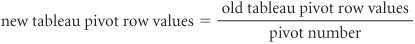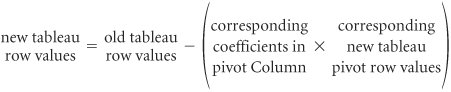# Summary of the Simplex Method

[Page A-16 ( continued )]

The simplex method demonstrated in the previous section consists of the following steps.

 1 Transform the model constraint inequalities into equations. 2 Set up the initial tableau for the basic feasible solution at the origin and compute the z j and c j z j row values. 3 Determine the pivot column (entering nonbasic solution variable) by selecting the column with the highest positive value in the c j z j row. 4 Determine the pivot row (leaving basic solution variable) by dividing the quantity column values by the pivot column values and selecting the row with the minimum nonnegative quotient . 5 Compute the new pivot row values using the formula6 Compute all other row values using the formula7 Compute the new z j and c j z j rows. 8 Determine whether or not the new solution is optimal by checking the c j z j row. If all c j z j row values are zero or negative, the solution is optimal. If a positive value exists, return to step 3 and repeat the simplex steps.Introduction to Management Science (10th Edition)
ISBN: 0136064361
EAN: 2147483647
Year: 2006
Pages: 358

Similar book on Amazon

flylib.com © 2008-2017.
If you may any questions please contact us: flylib@qtcs.net